UML软件工程组织

 结合 Ajax 进行 PHP 开发，第 1 部分: 入门一个简单的相册 Sean Kelly (skelly@idsociety.com), Web 应用程序开发人员, ID Society 　　来源：IBM

创建一个简单的相册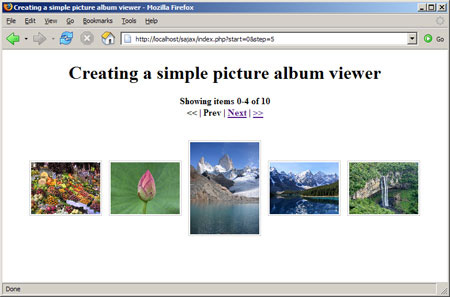首先要收集至少 20 幅 .jpg 图片，并将它们放到一个文件夹中。每个图片还要有一个保存在单独缩略图文件夹中的缩略图。虽然可使用 GD 软件包生成缩略图（请参阅 参考资料），但本文假设已经准备好了缩略图。也可使用本文提供的照片和缩略图（请参阅 下载）。

 /* * Find a list of images in /images and provide thumbnails */ function get_table ( \$limit_start = 0, \$limit_step = 5 ) { \$images = get_image_list('images'); // Generate navigation for Previous and Next buttons // Code given below \$output .= ''; \$columns = 5; foreach (\$images as \$index => \$image) { // Begin directory listing at item number \$limit_start if ( \$index < \$limit_start ) continue; // End directory listing at item number \$limit_end if ( \$index >= \$limit_start + \$limit_step ) continue; // Begin column if ( \$index - \$limit_start % \$columns == 0 ) { \$output .= '
' . get_image_link(\$thumbnail, \$index) . '
'; } // Generate link to blown up image (see below) \$thumbnail = ''; \$output .= ''; // Close column if ( \$index - \$limit_start % \$columns == \$columns - 1 ) { \$output .= ''; } } \$output .= ''; return \$nav . \$output; }

清单 2. get_file_list 实现

 function get_image_list ( \$image_dir ) { \$d = dir(\$image_dir); \$files = array(); if ( !\$d ) return null; while (false !== (\$file = \$d->read())) { // getimagesize returns true only on valid images if ( @getimagesize( \$image_dir . '/' . \$file ) ) { \$files[] = \$file; } } \$d->close(); return \$files; }

 // Append navigation \$output = '

Showing items ' . \$limit_start . '-' . min(\$limit_start + \$limit_step - 1, count(\$images)) . ' of ' . count(\$images) . '
'; \$prev_start = max(0, \$limit_start - \$limit_step); if ( \$limit_start > 0 ) { \$output .= get_table_link('<<', 0, \$limit_step); \$output .= ' | ' . get_table_link('Prev', \$prev_start, \$limit_step); } else { \$output .= '<< | Prev'; } // Append next button \$next_start = min(\$limit_start + \$limit_step, count(\$images)); if ( \$limit_start + \$limit_step < count(\$images) ) { \$output .= ' | ' . get_table_link('Next', \$next_start, \$limit_step); \$output .= ' | ' . get_table_link('>>', (count(\$images) - \$limit_step), \$limit_step); } else { \$output .= ' | Next | >>'; } \$output .= '

';

回页首

 function get_table_link ( \$title, \$start, \$step ) { \$link = "index.php?start=\$start&step=\$step"; return '' . \$title .''; } function get_image_link ( \$title, \$index ) { \$link = "expand.php?index=\$index"; return '' . \$title . ''; }

 function get_image ( \$index ) { \$images = get_image_list ( 'images' ); // Generate navigation \$output .= ''; return \$output; }

 \$output .= '

Viewing image ' . \$index . ' of ' . count(\$images) . '
'; if ( \$index > 0 ) { \$output .= get_image_link('<<', 0); \$output .= ' | ' . get_image_link('Prev', \$index-1); } else { \$output .= '<< | Prev'; } \$output .= ' | ' . get_table_link('Up', \$index, 5); if ( \$index < count(\$images) ) { \$output .= ' | ' . get_image_link('Next', \$index+1); \$output .= ' | ' . get_image_link('>>', count(\$images)); } else { \$output .= ' | Next | >>'; } \$output .= '

';

 Creating a simple picture album viewer

Creating a simple picture album viewer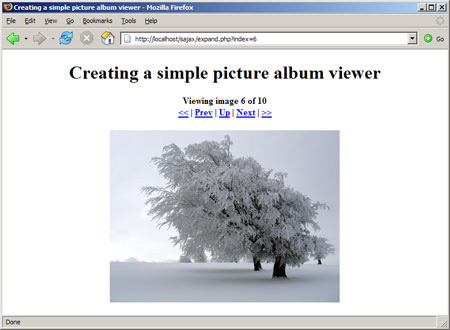添加 Sajax

Sajax、Ajax 与传统 Web 应用程序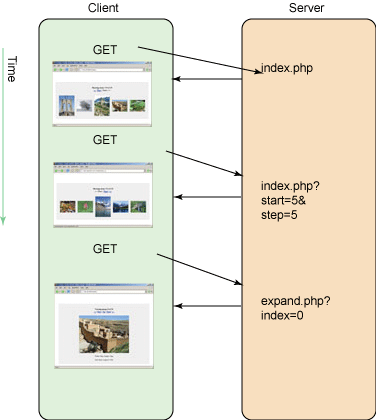Web 开发社区的人都知道，Ajax 允许向服务器发出异步的辅助请求，并直接在网页中显示结果（如图 4 所示）。不幸的是，即便最简单的 Ajax 应用程序实现起来也是一项大任务。因为 Ajax 不是标准化的技术，Internet Explorer 和其他浏览器（如 Firefox、Safari）的实现是不同的。此外，程序员至少要编写三个函数才能实现一个功能，这三个函数是：发送 HTTP 请求的初始 JavaScript，返回响应的 PHP 脚本，以及另一个处理这些响应的 JavaScript 函数。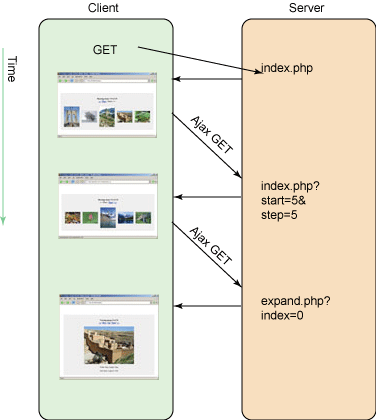建立在 Ajax 库之上的 Sajax 通过运用简单的启发式方法大大简化了这一过程：Web 客户机需要访问的每个 PHP 函数都由 Sajax “导出”。如果有一个名为 foo_bar() 的 PHP 函数，那么 Sajax 会把该函数导出为 JavaScript 函数 x_foo_bar()。客户机对 x_foo_bar() 的任何调用都会自动转发给服务器上的 foo_bar()，输出则传递给另一个 JavaScript 函数。清单 8 中的简短页面示范了这种功能。运行这个例子需要下载 Sajax 库（请参阅 参考资料）。

回页首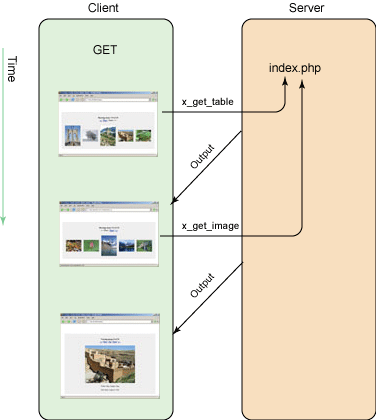将 Sajax 连接到相册





Sajax photo album

 Creating a Sajax photo album

图 6. 完成的基于 Sajax 的相册（缩略图）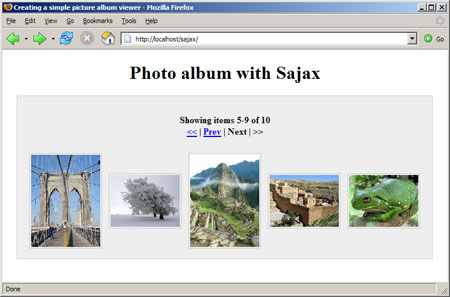可以看到 URL 仍然保持不变，并带来了更多愉快的用户体验。window div 显示在一个灰色的框中，通过 Sajax 生成的内容非常清楚。脚本不一定要知道自身或者它在服务器上的位置，因为所有的链接最终都成为直接对页面自身的 JavaScript 调用。因此我们的代码能够很好的模块化。我们只需要保持 JavaScript 和 PHP 函数在同一个页面上即可，即使页面位置发生了变化也没有关系。

回页首

 August 6, 2006 Los Angeles, CA Here's a photo of my favorite celebrity August 7, 2006 San Francisco, CA In SF, we got to ride the street cars August 8, 2006 Portland, OR Time to end our road trip!

 function get_meta_data ( \$file ) { // Using getimagesize, the server calculates the dimensions list(\$width, \$height) = @getimagesize("images/\$file"); \$output = "

Width: {\$width}px, Height: {\$height}px

"; // Use SimpleXML package in PHP_v5: // http://us3.php.net/manual/en/ref.simplexml.php \$xml = simplexml_load_file("gallery.xml"); foreach ( \$xml as \$photo ) { if (\$photo['id'] == \$file) { \$output .= !empty(\$photo->date) ? "

Date taken: {\$photo->date}

" : ''; \$output .= !empty(\$photo->locale) ? "

Location: {\$photo->locale}>/p>" : ''; \$output .= !empty(\$photo->comment) ? "

Comment: {\$photo->comment}

" : ''; } } return \$output;

 function get_image ( \$index ) { \$images = get_image_list ( 'images' ); // ... \$output .= ''; \$output .= '
' . get_meta_data( \$images[\$index] ) . '
'; return \$output; }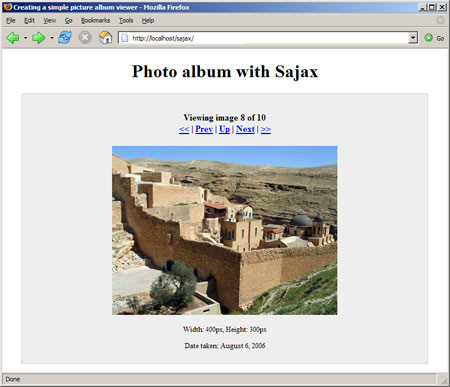结束语

 版权所有：UML软件工程组织